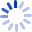# Discrete Invariants of Curves and Surfaces

by

First we discuss the construction of the signatures of curves and surfaces both in smooth and discrete cases. The purpose is to find appropriate differential and discrete invariants which can be used as coordinates in the pictures of signature curves and surfaces. Next we study variational problems for smooth curves and curves approximated by B-spline curves. Our main application is to the curve completion problem in 2D and 3D. In the smooth case, the aim is to find the solution of the smooth Euler-Lagrange equations which ...• Book Details
• Seller